# Quiz 10: Judgmental Forecasting and Forecast Adjustments

a. Compute the combined forecasts of sales produced by taking a simple average of the forecasts produced by Winter's method and the regression model. The general formula for combined forecasts using simple average method is given below: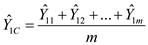Obtain the one-step-ahead combined forecasts using simple average method for the first month.Thus, the one-step-ahead combined forecasts using simple average method for the first month is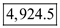. Obtain the one-step-ahead combined forecasts using simple average method for the second month.Thus, the one-step-ahead combined forecasts using simple average method for the second month is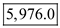. Obtain the one-step-ahead combined forecasts using simple average method for the third month.Thus, the one-step-ahead combined forecasts using simple average method for the third month is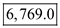. Similarly, the combined forecasts of sales produced by Winter's method and the regression method can be calculated. The results of the combined forecasts for all months are tabulated below: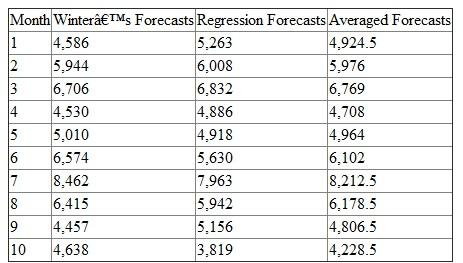b. Compute the combined forecasts of sales produced by taking a weighted average of the forecasts produced by Winters' method and the regression model. The general formula for combined forecasts using weighted average is obtained below: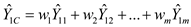Obtain the one-step-ahead combined forecasts using weighted average method for the first month.Thus, the one-step-ahead combined forecasts using weighted average method for the first month is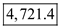. Obtain the one-step-ahead combined forecasts using weighted average method for the second month.Thus, the one-step-ahead combined forecasts using weighted average method for the second month is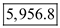. Obtain the one-step-ahead combined forecasts using weighted average method for the third month.Thus, the one-step-ahead combined forecasts using weighted average method for the third month is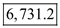. Similarly, the combined forecasts of sales produced by Winters' method and the regression method can be obtained. The results of the combined forecasts for all months are tabulated below: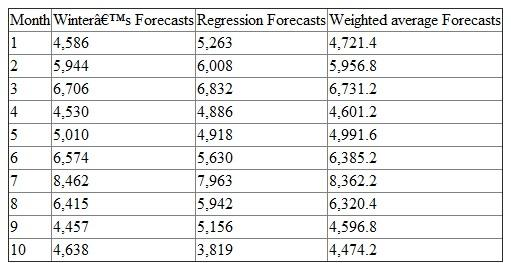c. Compute the Mean Absolute Percentage Error ( MAPE ) for the Winters' forecasting method.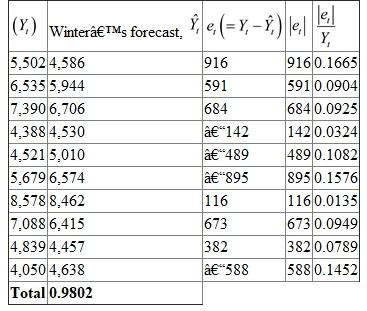The value of the Mean Absolute Percentage Error ( MAPE ) is,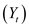Thus, the Mean Absolute Percentage Error ( MAPE ) for the Winter's forecasting method is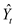. Compute the Mean Absolute Percentage Error ( MAPE ) for the regression forecasting method.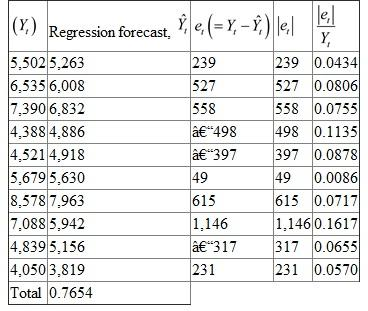The value of the Mean Absolute Percentage Error ( MAPE ) is,Thus, the Mean Absolute Percentage Error ( MAPE ) for the regression forecasting method is. d. Compute the Mean Absolute Percentage Error ( MAPE ) for the average forecasting method.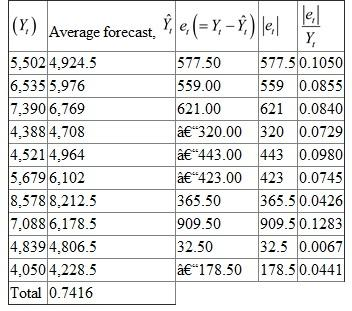The value of the Mean Absolute Percentage Error ( MAPE ) is,Thus, the Mean Absolute Percentage Error ( MAPE ) for the average forecasting method is. Compute the Mean Absolute Percentage Error ( MAPE ) for the weighted average forecasting method.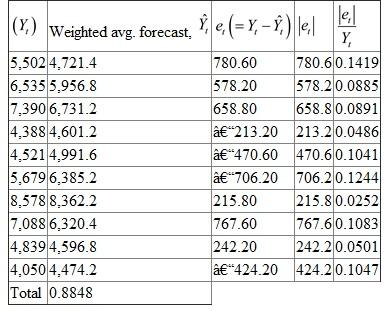The value of the Mean Absolute Percentage Error ( MAPE ) is,Thus, the Mean Absolute Percentage Error ( MAPE ) for the weighted average forecasting method is. Identify the preferable forecasts method based on the Mean Absolute Percentage Error ( MAPE ) measure. From part (b) and (c), it is clear that the simple average and regression forecasts methods are preferable, because the error measures by simple average forecasts and regression forecasts is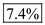and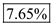is comparatively lesser than the other forecasts methods.